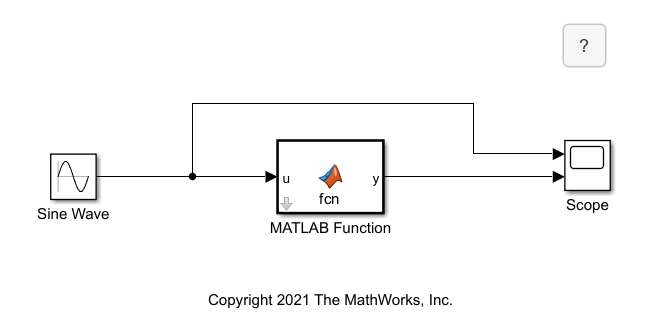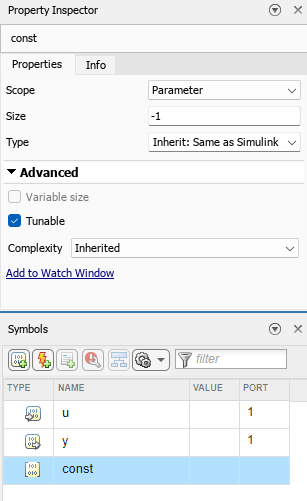## Configure MATLAB Function Block Parameter Data Arguments

You can use parameter data arguments in MATLAB Function blocks to use variables defined in the MATLAB® workspace, model workspace, a masked subsystem workspace, or a Simulink® data dictionary as input arguments. Simulink searches for and selects variables used by parameter data arguments by using the same rules for symbol resolution. See Symbol Resolution. If a variable of the same name exists in more than one of the workspaces visible to the block, the block uses the variable closest to the block in the workspace hierarchy. If the MATLAB Function block is in a masked subsystem, the block uses the variable in the subsystem workspace. Otherwise, the block uses the variable in the model workspace, followed by using the variable in the MATLAB base workspace and in an associated Simulink data dictionary, in that order.

To specify parameter data arguments, in the Model Explorer or the Ports and Data Manager, create or select existing data and set the Scope property to `Parameter`. For more information about creating arguments and data in MATLAB Function blocks, see Define MATLAB Function Block Data. Parameter data arguments do not create block ports.

### Control Parameter Data Arguments with Masks

You can directly assign values to parameter data arguments in a MATLAB Function block by adding a mask to the block, creating a parameter in the mask, and giving the parameter the same name as the parameter data argument. For more information about creating masks for blocks, see Masking Fundamentals. After you mask the block, you can adjust the parameter data argument properties by using the Ports and Data Manager, but not the Model Explorer.

#### Adjust Parameter Data Argument Example

This example uses a masked MATLAB Function block with a parameter data argument.The MATLAB function multiplies the parameter data argument `const` by the value of the Sine Wave block signal. Click the arrow icon on the MATLAB Function block to view the code.

```function y = fcn(u, const) ```
```y = const*u; ```

You can adjust the value of `const` by double-clicking the MATLAB Function block. The block mask defines the Set sine wave multiplier parameter, which you can edit in the Mask Editor. The MATLAB Function block defines `const` as data with Scope set to `Parameter`.The parameter data argument and the mask parameter must have the same name. In this example, changing the parameter data argument name without changing the parameter name causes an error.

### Control Parameter Data Arguments in Workspaces

If you want multiple MATLAB Function blocks to access the same variable in a model, you can define the variable in the model workspace and add parameter data arguments to each MATLAB function that points to that variable. You can define the variable directly in the model workspace, load the variable in a MAT-file or MATLAB file, or create the variable with custom MATLAB code. For more information, see Specify Source for Data in Model Workspace.

If you want to use the variable in MATLAB Function blocks in multiple models, you can define the variable in the MATLAB base workspace by defining the variable in the Command Window or by loading a MAT-file. MATLAB Function blocks access variables defined in the MATLAB workspace until you close MATLAB.

You can also define variables using a Simulink data dictionary. For more information, see What Is a Data Dictionary?.